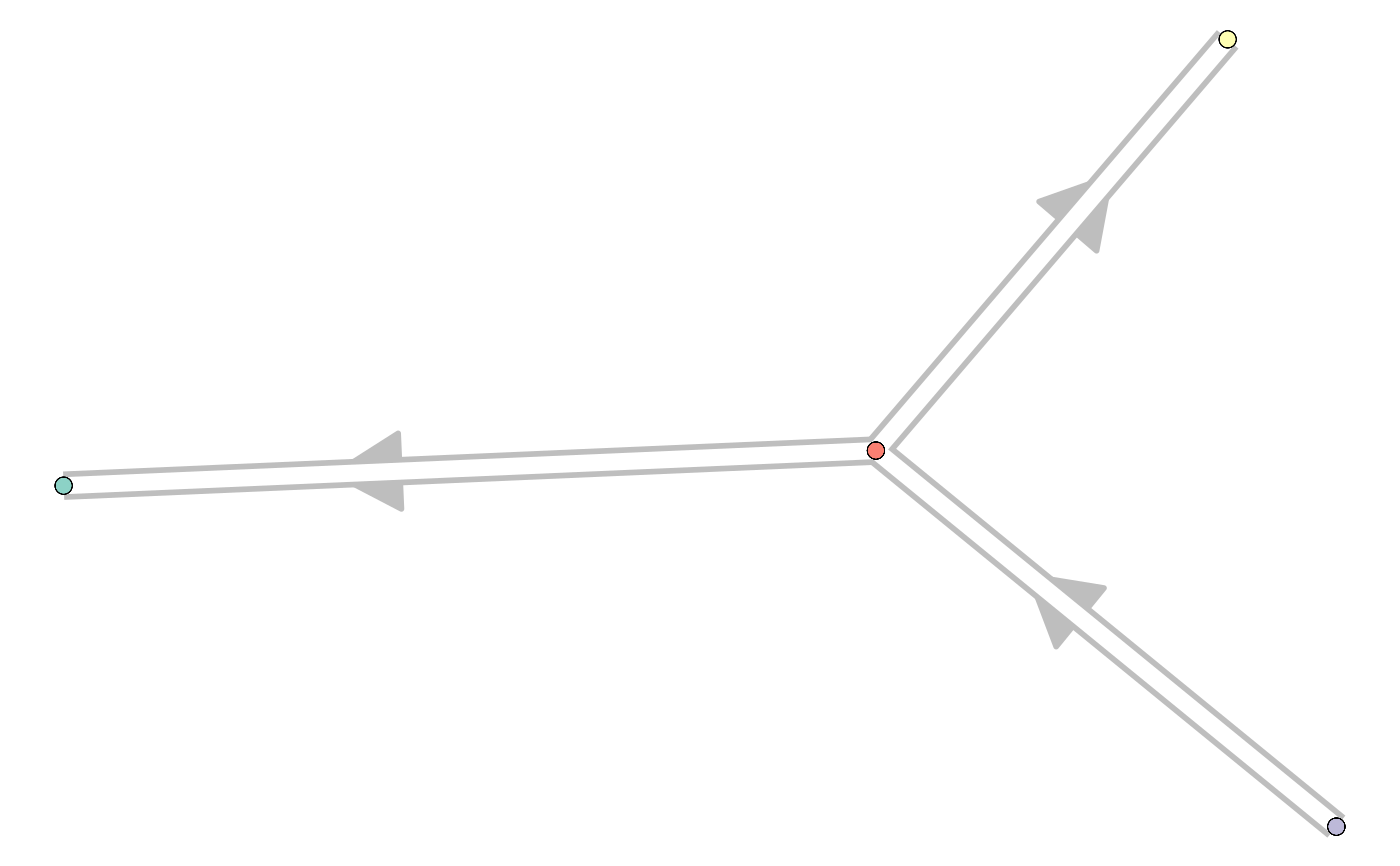# Constructs a trajectory using a cell grouping and a network between groups. Will use an existing grouping if it is present in the dataset.

This function will generate the milestone_network and progressions.

```add_cluster_graph(dataset, milestone_network, grouping = NULL,
explicit_splits = FALSE, ...)```

## Arguments

dataset A dataset created by `wrap_data()` or `wrap_expression()` A network of milestones. A grouping of the cells, can be a named vector or a dataframe with group_id (character) and cell_id (character) Whether to make splits specific by adding a starting node. For example: A->B, A->C becomes A->X, X->B, X->C extra information to be stored in the wrapper.

The trajectory

## Examples

```library(tibble)
dataset <- wrap_data(cell_ids = letters)

milestone_network <- tibble::tibble(
from = c("A", "B", "B"),
to = c("B", "C", "D"),
directed = TRUE,
length = 1
)
milestone_network#> # A tibble: 3 x 4
#>   from  to    directed length
#>   <chr> <chr> <lgl>     <dbl>
#> 1 A     B     TRUE          1
#> 2 B     C     TRUE          1
#> 3 B     D     TRUE          1grouping <- sample(c("A", "B", "C", "D"), length(dataset\$cell_ids), replace = TRUE)
grouping#>   "B" "D" "B" "A" "A" "B" "A" "D" "C" "B" "A" "C" "D" "A" "A" "D" "A" "A" "D" "B" "B" "A" "B" "C" "C" "B"trajectory <- add_cluster_graph(dataset, milestone_network, grouping)

# for plotting the result, install dynplot
dynplot::plot_graph(trajectory)#> Coloring by milestone#> Using milestone_percentages from trajectory```